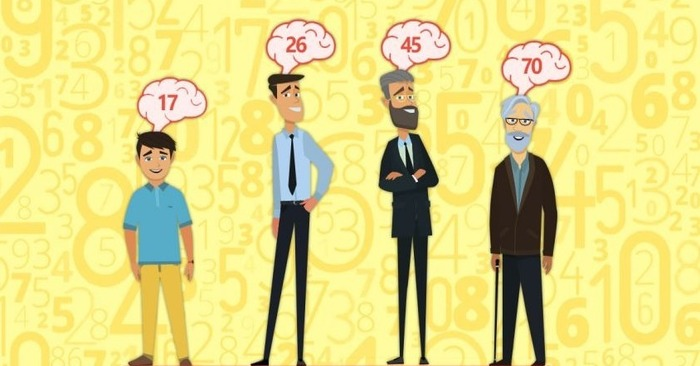# This mathematical test will disclose your age with 100 percent accuracyThis simple but effective test will reveal your real age

Did you know the fact you can define person’s real age with the help of a simple mathematical test? Don’t you believe? Then, we offer you to take this mathematical test right now.

1. To start with, choose one of the numerals: 1,2,3,4,5,6,7,8,9. The numeral you choose will be denoted as X.
2. Now you should multiply it by two. X * 2.
3. Add 5. (X * 2) + 5.
4. Multiply the final result by fifty. (X * 2 + 5) * 50.
5. If this year you celebrated your birthday, then you should add 1767 to the result. If not, then add 1766.
6. Now from the last result you got it is necessary to subtract the year you were born.